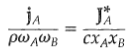### Create an Account

Home / Questions / Relations between fluxes in binary systems the following equation is useful for interrela...

# Relations between fluxes in binary systems the following equation is useful for interrelating expressions in mass units and those in molar units in two component systems Verify the correctness of

Relations between fluxes in binary systems, the following equation is useful for interrelating expressions in mass units and those in molar units in two-component systems: Verify the correctness of thisrelation.Jul 25 2020 View more View LessSubscribe To Get Solution